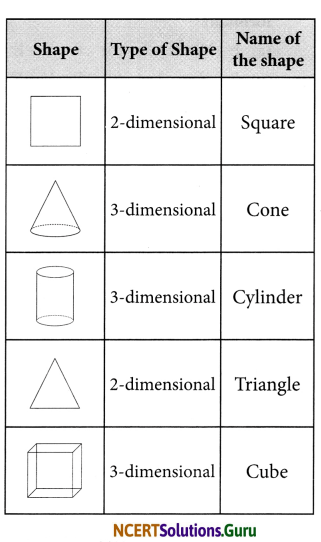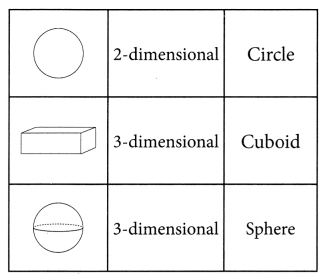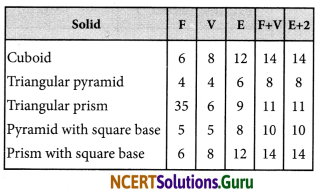# NCERT Solutions for Class 8 Maths Chapter 10 Visualizing Solid Shapes InText Questions

These NCERT Solutions for Class 8 Maths Chapter 10 Visualizing Solid Shapes InText Questions and Answers are prepared by our highly skilled subject experts.

## NCERT Solutions for Class 8 Maths Chapter 10 Visualizing Solid Shapes InText Questions

NCERT Intext Question Page No. 153
Question 1.
Match the following: (First one is done for you)Question 2.
Match the following pictures (objects) with their shapes:(i) A triangular field adjoining a square field.
(ii) A cone taken out of a cylinder.
(iii) A hemisphere surmounted on a cone.
(iv) A circular path around a circular ground.
(v) Two rectangular cross paths inside a rectangular park.NCERT Intext Question Page No. 165
Question 1.
Tabulate the number of faces, edges and vertices for the following polyhedrons: (Here ‘V’ stands for number of vertices, ‘F’ stands for number of faces and ‘E’ stands for number of edges).